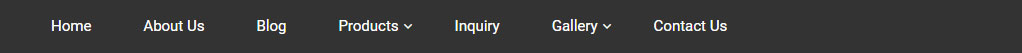Mathematics#### "   અમો કારકીર્દીનું  નિર્માણ કરીયે છીએ   "Home / Mathematics### Mathematics

The history of mathematics is nearly as old as humanity itself 3000 years ago the Greeks started to look for rational explanations for natural phenomena and the laid the foundation for geometry and arithmetic.

The most ancient mathematical texts from Mesopotamia and Egypt are from 2000-1800 BC many early texts mention Pythagorean triples and so by inference, the Pythagorean theorem seems to be the most ancient and widespread mathematical development after basic arithmetic and geometry around 300 BC Euclide introduce the axiomatic method still used in mathematic today consisting of definition axiom theorem and proof.

In India mathematics emerged in the Indian subcontinent from 1200 BC until the end of the 18th century important contributions were made by like Aryabhata, Brahmagupta, Bhaskaracharya and Varahmihira. The decimal number system in use today was first recorded in Indian mathematics.

Indian mathematicians made early contribution to the study of the concept of zero as a number negative numbers, arithmetic and algebra. In addition trigonometry was further advanced in India and in particular the modern definitions if sine and cosine were developed there. These mathematical concepts were transmitted to the Middle East, China and Europe and led to further developments that now form the foundations of many areas of mathematics.

Ancient and medieval Indian mathematical works all composed in Sanskrit usually consisted of a section of sutras in which a set of rules or problems were stated with great economy in verse in order to aid memorization by a student.